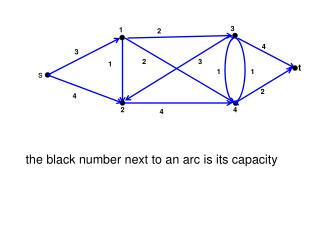DownloadDownload Presentation4

# 4

Télécharger la présentation## 4

- - - - - - - - - - - - - - - - - - - - - - - - - - - E N D - - - - - - - - - - - - - - - - - - - - - - - - - - -
##### Presentation Transcript

1. 3 1 2 4 3 2 3 1 t 1 1 s 2 4 2 4 4 the black number next to an arc is its capacity

2. 3 1 2 4 3 2 3 1 t 1 1 s 2 4 2 4 4 Cts= -1 Set costs all other arcs at 0 The minimum cost flow circulation (Af=0) maximises the s-t flow

3. 3 1 2 4 3 2 3 1 t 1 1 s 2 4 2 4 4 the black number next to an arc is its capacity

4. 3 1 11 2 22 4 3 3 2 2 3 3 the residual graph 1 1 t 1 1 1 1 s 2 2 13 4 2 4 13 4 blue arcs (i,j) are forward arcs (fij<uij) green arcs (j,i) are backward arcs (fij>0) the blue number is the residual capacity of a blue arc the green number is the capacity of a green arc the black number is the original capacity of the arc

5. 3 1 11 22 3 2 3 the residual graph 1 t 1 1 s 2 13 2 4 13 red arcs form an augmenting path

6. 3 1 11 22 3 2 3 the residual graph 1 t 1 1 s 2 13 2 4 13 red arcs form an augmenting path Augment the flow by the minimum capacity of a red arc, i.e, 1

7. 3 1 2 3 1 3 3 the residual graph 1 t 11 1 1 s 2 22 2 4 22 red ars form an augmenting path Augment the flow by the minimum capacity of a red arc, i.e, 1 And construct the new residual graph

8. 3 1 2 4 3 2 3 1 t 1 1 s 2 4 2 4 4 An s-t cut is defined by a set S of the nodes with s in S and t not in S.

9. 3 1 2 4 3 2 3 1 t 1 1 s 2 4 2 4 4 S={s,1,2} An s-t cut is defined by a set S of the nodes with s in S and t not in S.

10. 3 1 2 4 3 2 3 1 t 1 1 s 2 4 2 4 4 S={s,1,2} An s-t cut is defined by a set S of the nodes with s in S and t not in S Size of cut S is the sum of the capacities on the arcs from S to N\S.

11. 3 1 2 4 3 2 3 1 t 1 1 s 2 4 2 4 4 C(S)=u13+u14+u24= 2+2+4=8 An s-t cut is defined by a set S of the nodes with s in S and t not in S Size of cut S is the sum of the capacities on the arcs from S to N\S.

12. S1 3 1 2 4 3 2 3 1 t 1 1 s 2 4 2 4 4 C(S1)=us1+u24= 3+4=7

13. S2 S1 3 1 2 4 3 2 3 1 t 1 1 s 2 4 2 4 4 C(S1)=us1+u24= 3+4=7 C(S2)=u13+u43+u4t= 2+1+2=5

14. S2 S1 3 1 2 4 3 2 3 1 t 1 1 s 2 4 2 4 4 C(S1)=us1+u24= 3+4=7 C(S2)=u13+u43+u4t= 2+1+2=5 Max Flow ≤ Min Cut

15. S2 S1 3 1 2 4 3 2 3 1 t 1 1 s 2 4 2 4 4 C(S1)=us1+u24= 3+4=7 C(S2)=u13+u43+u4t= 2+1+2=5 Max Flow ≤ Min Cut fs1+fs2 ≤ Min Cut ≤ 5

16. S2 1 2 3 1 3 3 the residual graph 1 t 11 1 1 s 2 22 2 4 22 = fs1+fs2=2+3=5 C(S2)=u13+u43+u4t= 2+1+2=5 S2={s,1,2,4} the set of nodes reachable from S Max Flow ≤ Min Cut

17. S2 1 2 3 1 3 3 the residual graph 1 t 11 1 1 s 2 22 2 4 22 = fs1+fs2=2+3=5 C(S2)=u13+u43+u4t= 2+1+2=5 S2={s,1,2,4} the set of nodes reachable from S Max Flow ≤ Min Cut Theorem: Max Flow = Min Cut